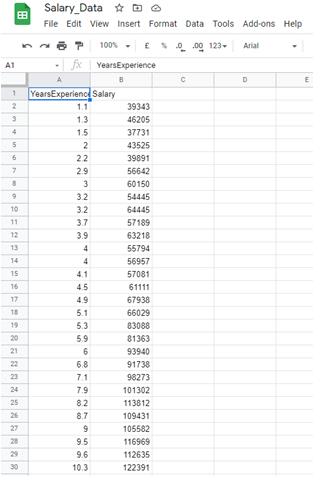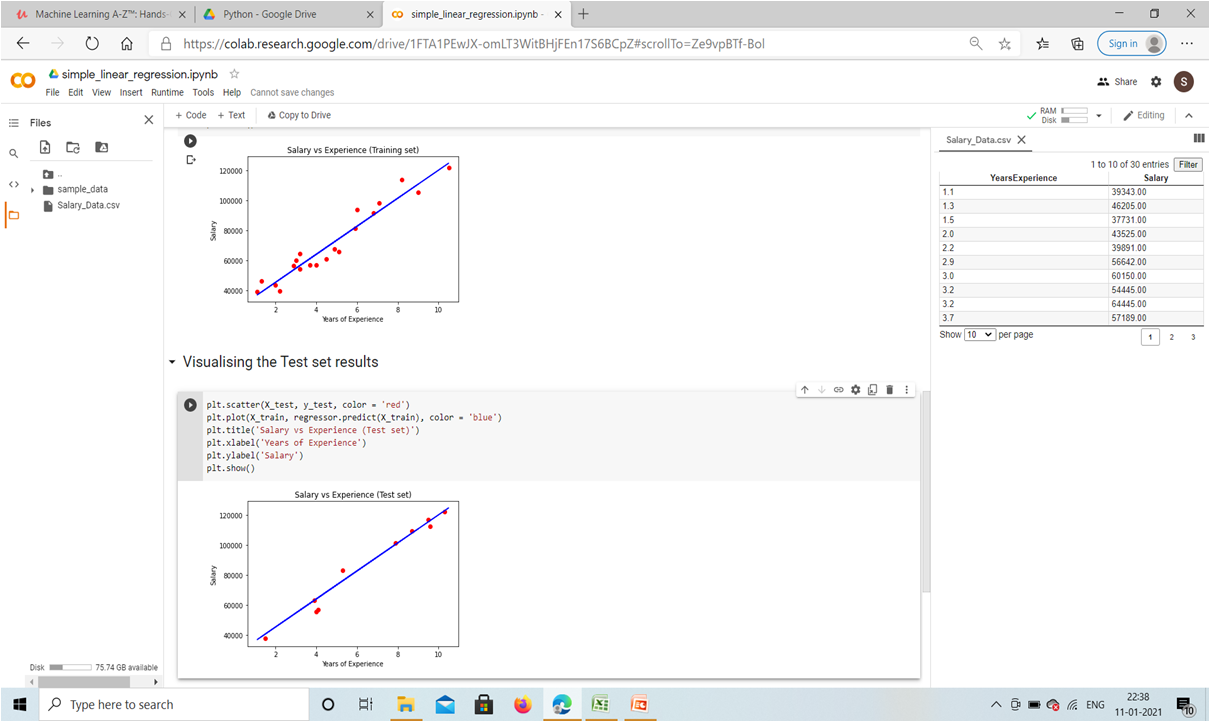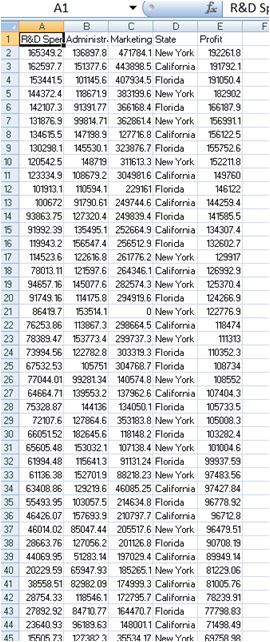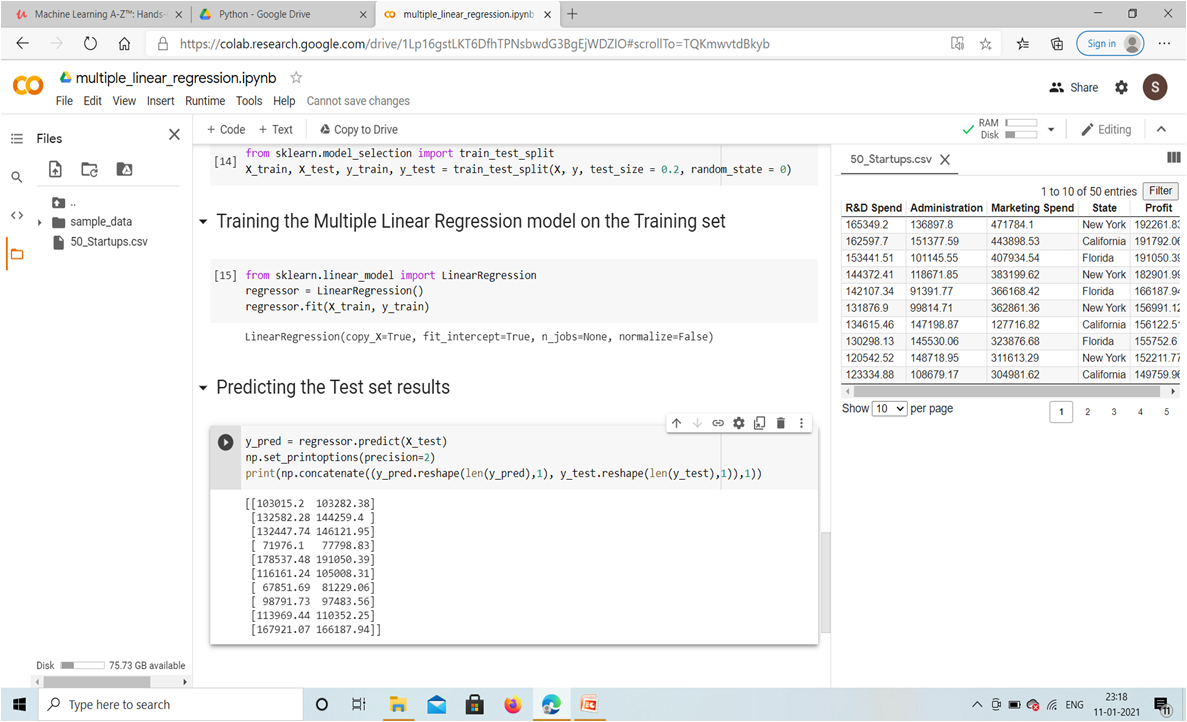# Customer Purchasing Behaviour

Last Updated on May 3, 2021

## Now, they want to build a model to predict the purchase amount of customer against various products which will help them to create personalized offer for customers against different products.

More Details: Customer Purchasing Behaviour
###### Share with someone who needs it# Regression Model

Last Updated on May 3, 2021

# Regression

It is a supervised technique, regression models are used to predict a continuous value, the goal of regression model is to build a mathematical equation that defines y as a function of the x variables.

Y = mX + B , where X is independent variable and Y is dependent variable.

}Eg; Salary of employee based on years of experience. In equation (Y = mX + B) , Y is salary, m is slope, X is years of experience, B is y intercept.

TYPES OF REGRESSION

• Simple Linear Regression
• Multiple Linear Regression
• Polynomial Linear Regression
• Support Vector Regression(SVR)
• Decision Tree Regression
• Random Forest Regression

Table of contents

• Importing the libraries:
• Numpy for working with arrays, linear algebra, Matplotlip for plotting using its module pyplot , Pandas for data manipulation and analysis.
• Importing the dataset:

Create a new variable (dataset) , call certain functions from pandas library . Dataset = Pd.read_csv(‘data.csv’).

• Taking care of missing data:

Using Scikit-learn it is a library that provides many unsupervised and supervised learning algorithms. By using imputer we are filling the missing data by average of the column. Fit-method identifies the missing data and calculate mean, Transform-method will replace the missing by average salary.

• Encoding Categorical Data:

Using one hot encoding it is a representation of categorical variables as binary vectors using integers.

• Splitting the dataset into the Training set and Test set:

Training set = training ML model on existing observations.

Test set = performance on new observations

Creating 2 entities Y(independent) salary, X (dependent) years of experience.

• Feature scaling:

it is performed so that some features don’t dominate other features. It is done after splitting to prevent data leakage, from sklearn model selection is used which creates 4 separate sets i.e 2 for training, 2 for test.

• 2 techniques of feature scaling are : standardization, normalization.
• Training the model on the Training set
• Predicting the Test set results
• Visualizing the Training set results
• Visualizing the Test set results

## Open Source Project Of a Real Life Example

}1) Simple Linear Regression

}Problem description: Dataset with 30 observations, goal is to build a simple linear regression model to understand correlation btw years of experience and salary to predict the salary for new employee with specific years of experience.

Table of contents:

}Importing the libraries

}Importing the dataset

}Splitting the dataset into the Training set and Test set

}Training the Simple Linear Regression model on the Training set

}Predicting the Test set results

}Visualizing the Training set results: in graph the regression line is as close as the actual salaries.

}Visualizing the Test set results: the predicted salaries are close to actual salaries .

The dataset:RESULT2) Multiple Linear Regression

}Problem description: IN Data set of 50 start-ups, one should invest in which company to achieve the goal of maximizing profit .

}Table of contents:

}Multiple Linear Regression

}Importing the libraries

}Importing the dataset

}Encoding categorical data: using one hot encoding (Yew York = 001, California = 100, Florida = 010)

}Splitting the dataset into the Training set and Test set

}Training the Multiple Linear Regression model on the Training set

}Predicting the Test set results: test set has 20% of whole dataset so 20% of 50 startups = 10 observations ; we get 2 vectors

}1st is of 10 real profit from test set , 2nd 10 predicted profit of same.

}Then concatenate (from numpy concatenate 2 vectors predicted, real )

}In the result on the left we have the first vector = predicted profit (Y_pred), on the right we have secound vector = real profit (Y_test).

}Hence MLR is well adapted to this dataset

The dataset:RESULTPolynomial Linear Regression

}Problem description: 10 observations in the dataset. The previous salary mentioned by candidate is 16k Goal is to predict the previous salary of the candidate also the present salary to be provided according to the post applied so that we know the salary mentioned by candidate is bluff or true.

}Table of contents:

}Importing the libraries

}Importing the dataset

}Training the Linear Regression model on the whole dataset

}Training the Polynomial Regression model on the whole dataset

}Visualizing the Linear Regression results: on graph red is the real salary and blue is regression line with predictions, linear model is not adapted as far from prediction.

}Visualizing the Polynomial Regression results: better result as the greaater the degree the better is the prediction.

}Visualizing the Polynomial Regression results (for higher resolution and smoother curve): increase the number of points on x axis.

}Predicting a new result with Linear Regression: predicted salary is 330378.78 >16k hence bad prediction.

}Predicting a new result with Polynomial Regression: : predicted salary is 158862.45 approx 16 so acceptable.

The dataset: